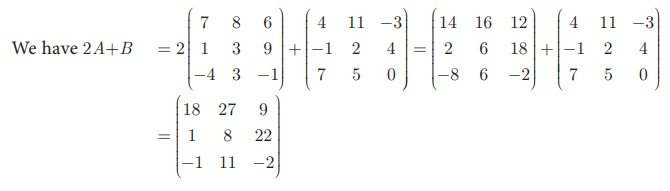Home | | Maths 10th Std | Multiplication of Matrix by a Scalar

# Multiplication of Matrix by a Scalar

We can multiply the elements of the given matrix A by a non-zero number k to obtain a new matrix kA whose elements are multiplied by k. The matrix kA is called scalar multiplication of A.

## Multiplication of Matrix by a Scalar

We can multiply the elements of the given matrix A by a non-zero number k to obtain a new matrix kA whose elements are multiplied by k. The matrix kA is called scalar multiplication of A.

Thus if A = (aij )m ×n  then , kA = (kaij )m ×n  for all i = 1,2,…,m and for all j = 1,2,…,n.

### Example 3.60

If A =then Find 2A+B.

### Solution

Since A and B have same order 3 ×3 , 2A + B is defined.Example 3.61Solution

Since A, B are of the same order 3 ×3 , subtraction of 4A and 3B is defined.Study Material, Lecturing Notes, Assignment, Reference, Wiki description explanation, brief detail
10th Mathematics : UNIT 3 : Algebra : Multiplication of Matrix by a Scalar |